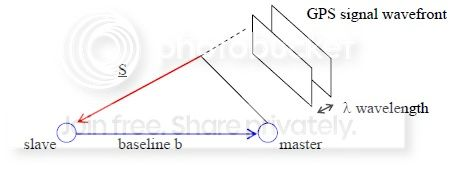# How does GPS work exactly?

For a GPS receiver to estimate its position, it first receives signals from at least 4 satellites. Does the receiver calculate the distance that separates it from each satellite, or does it just calculate the distance difference between each pair of satellites?

In other words, does the GPS system use TOF (time of flight) or TDOA (time difference of arrival, aka multilateration) technique to calculate position and how are these techniques applied?

I think GPS receivers do not exclusively need at least 4 satellites, but rather 3 as a minimum for trilateration.A fourth satellite signal is necessary to synchronize the receiver clock with the satellite clocks.

As for TOF/TOA vs. TDOA, I believe the difference relies whether on if the GPS receiver has an internal clock synchronized with the satellite transmitters or not. In other words, whether if you know the time on the satellite or not (in which case, you measure the range differences).

So, user-end GPS systems probably use TDOA as they wouldn't have a synchronized clock (would be too expensive).

Details on the GPS positioning calculations:The GPS calculation in the receiver uses four equations in the four unknowns x, y, z, tc, where x, y, z are the receiver's coordinates, and tc is the time correction for the GPS receiver's clock.

The equation is:

\begin{equation} \ d_n = c(t_{t,n} - t_{r,n} + t_c) = \sqrt[]{(x_n-x)^2+(y_n-y)^2+(z_n-z)^2} \end{equation}

Where:

• n would be: GPS 1,2,3,4 (for each of the 4 equations respectively)
• c = speed of light (3x108 m/s)
• tt,1, tt,2, tt,3, tt,4 = times that GPS satellites 1, 2, 3, and 4, respectively, transmitted their signals (these times are provided to the receiver as part of the information that is transmitted).
• tr,1, tr,2, tr,3, tr,4 = times that the signals from GPS satellites 1, 2, 3, and 4, respectively, are received (according to the inaccurate GPS receiver's clock)
• x1, y1, z1 = coordinates of GPS satellite 1 (these coordinates are provided to the receiver as part of the information that is transmitted); similar meaning for x2, y2, z2, etc.

TOA = Time of arrival, TOF = Time of flight, TDOA = Time differences of arrival

• Thanks for the answer, this is what I attempted to confirm, because as you said, without synchronization between the sender and the satellites, it is not possible to get TOF measurement. In the other hand, because satellites are perfectly synchronized between them, TDOA is the only possible way to calculat the receiver position – Noureddine Jan 13 '15 at 10:46
• In theory a generic Satellite Navigation System could operate with 3 satellites if we could be sure that all timings are totally accurate, but in practice GPS/Glonass/Galileo all use 4, which is both more accurate/reliable, but also easier to implement. See this question gis.stackexchange.com/q/12866 - the important point is that while the satellites are perfectly synchronised, the receiver is not guaranteed to be. – Jon Story Jan 13 '15 at 16:13
• Thanks for the link @JonStory it looks like I am a bit wrong about the 4th satellite's function according to it. Telecoms is not my field of expertise and what I am stating here is what little I recall from some lectures I attended while studying CS. I'll try refine my answer with a bit of research. It would help though when someone marks the answer as wrong/not useful, to add a comment on why, I think that would be constructive. – CodingDuckling Jan 14 '15 at 8:53
• @CodingDuckling yeah I always find that the biggest problem on SE - blind down voting or flagging is ok for 'bad' posts, but is counter productive for 'right apart from xyz detail which needs improving'. Your basic theory was right, though, the systems could work with 3 satellites in the way you describe – Jon Story Jan 14 '15 at 9:21
• Thanks for all your adds and comments, I think that we need 4 satellites as we are working in 3D, where the intersection of four spheres lead to one point. However, my main question is about the way that GPS calculates the distance, which is not clearly mentioned. – Noureddine Jan 14 '15 at 17:31

GPS satellites can be used to determine the attitude of other satellites also. I am not an expert in this field, but it might work the same way for tracking of people/cars on Earth.

Anyway, this is how it works for satellites.GPS sensors can be used to determine attitude provided that at least 3 antennas are vailable. The 4th is needed for timing.

Slave and master are 2 antennas mounted on the s/c. If the distance $b$ is known we can detect the s/c orientation in space. Taking the projection of $\underline{b}$ on the direction $\underline{S}$ of the incoming GPS signal we obtain the path difference of the signal received by the 2 antennas as $\underline{S}^T \underline{b}$.

The position of the GPS satellite is known, therefore $\underline{S}$ is known in the geocentric reference frame if we know the position of the master antenna.

Measuring the path difference

$\Delta r = \underline{S}^T \underline{b}$

and transforming the vector $\underline{S}$ from geocentric to body frame

$\underline{S} = AS$

$\Delta r = S^T A^T \underline{b}$

the unknown is the rotation matrix A.

Having 3 independent measurements:

$\Delta r_{11} = S_1^T A^T \underline{b}_1$ baseline 1 GPS satellite 1

$\Delta r_{21} = S_2^T A^T \underline{b}_1$ baseline 1 GPS satellite 2

$\Delta r_{12} = S_1^T A^T \underline{b}_2$ baseline 2 GPS satellite 1

$\Delta r_{22} = S_2^T A^T \underline{b}_2$ baseline 2 GPS satellite 2

...

2 baselines are required, otherwise if you have a single baseline you cannot determine the rotation around that baseline.

To determine attitude minimize the cost function:

$J = \sum_{i = 1}^{N_S} \sum_{j = 1}^{N_b} (\Delta r_{ij} - S_i^T A^T b_j)$